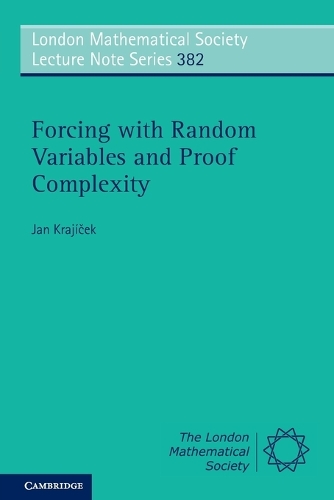•# Forcing with Random Variables and Proof Complexity - London Mathematical Society Lecture Note Series (Paperback)

(author)
£50.99
Paperback Published: 23/12/2010
• We can order this from the publisher

UK delivery within 4-5 weeks

This book introduces a new approach to building models of bounded arithmetic, with techniques drawn from recent results in computational complexity. Propositional proof systems and bounded arithmetics are closely related. In particular, proving lower bounds on the lengths of proofs in propositional proof systems is equivalent to constructing certain extensions of models of bounded arithmetic. This offers a clean and coherent framework for thinking about lower bounds for proof lengths, and it has proved quite successful in the past. This book outlines a brand new method for constructing models of bounded arithmetic, thus for proving independence results and establishing lower bounds for proof lengths. The models are built from random variables defined on a sample space which is a non-standard finite set and sampled by functions of some restricted computational complexity. It will appeal to anyone interested in logical approaches to fundamental problems in complexity theory.

Publisher: Cambridge University Press
ISBN: 9780521154338
Weight: 380 g
Dimensions: 228 x 152 x 15 mm

MEDIA REVIEWS
"Jan Krajíček is the leading expert on these problems and in this book he provides a new approach to builing models of bounded arithmetic which combines methods and techniques from model theory, forcing and computational complexity. Personally, I find Krajíček's approach a highly stimulating collage of ideas. I recommend this book strongly to anyone interested in logical approaches to fundamental problems in complexity theory." Soren M. Riis for Mathematical Reviews